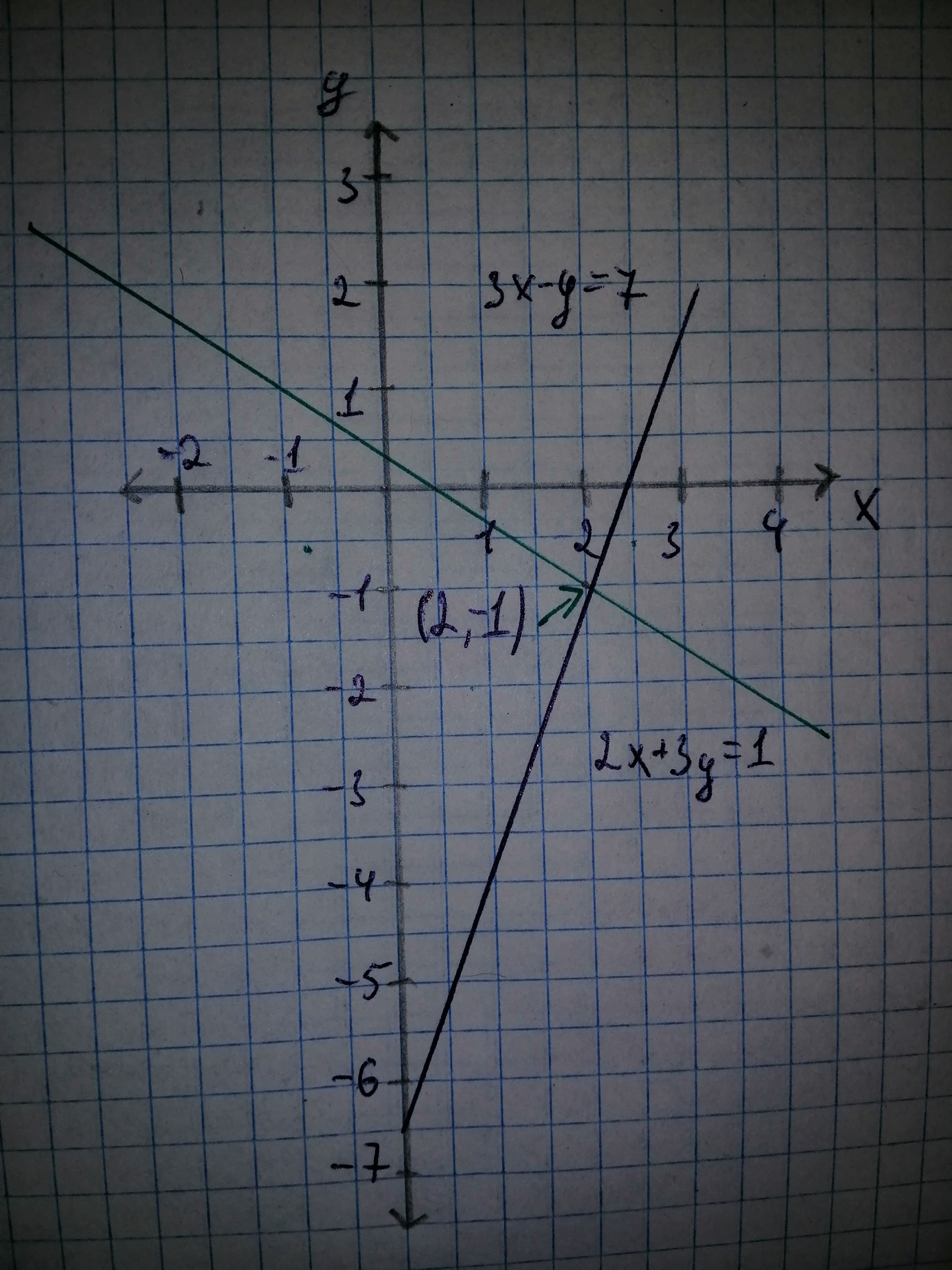# As you can see the solution to the system is the coordinates of the point where the two lines intersect. So, when solving linear systems with two variables we are really asking where the two lineas will intersect.necessaryh 2020-12-02 Answered
As you can see the solution to the system is the coordinates of the point where the two lines intersect. So, when solving linear systems with two variables we are really asking where the two lineas will intersect.
You can still ask an expert for help

• Questions are typically answered in as fast as 30 minutes

Solve your problem for the price of one coffee

• Math expert for every subject
• Pay only if we can solve itnitruraviX

A linear system of two equations with two variables is any system that can be written in the form.

Where any of the constants can be zero with the exception that each equation must have at least one variable in it.
Also, the system is called linear if the variables are only to the first power, are only in the numerator and there are no products of variables in any of the equations.
Here is an example of a system with numbers.

Before we discuss how to solve systems we should talk about just what a solution to a system of equations is.
A solution to a system of equations is a value of x and a value of y that, when substituted into the equations, satisfies both equations at the same time.
For the example above is a solution to the system. well if you think about it both of the equations in the system are lines. So, let's graph them and see what we get.As you can see the solution to the system is the coordinates of the point where the two lines intersect. So, when solving linear systems with two variables we are really asking where the two lines will intersect.